# AC (Alternating Current) Generators

AC-Circuits > AC (Alternating Current) Generators

An Electrical Generator is a device that produces an Electromotive Force (e.m.f.) by changing the number of Magnetic Flux Lines (Lines of Force), Φ, passing through a Wire Coil. Figure 1 is one type of Generators. When the Coil is rotated between the Poles of the Magnet by cranking the handle, an AC Voltage Waveform is produced.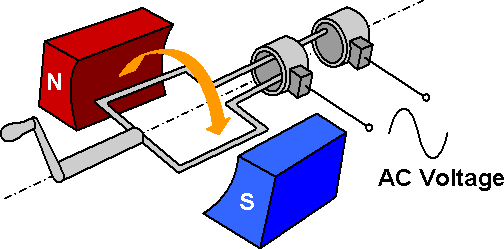Figure 1: Electrical Generator

Operation principle of a Generator is based on Electromagnetic Induction, which is defined by Faraday's Law, which states: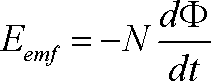The Electromotive Force, Eemf, induced in a Coil is proportional to the number of turns, N, in the Coil and the Rate of Change, dΦ / dt, of the number of Magnetic Flux Lines, Φ, passing through the surface (A) enclosed by the Coil.

An Induced Effect is always such as to Oppose the cause that produced it.

In the Generator, the Coil is under a Stationary Magnetic Field. The Magnetic Flux Density, B, is constant and Φ = B x Aeff, so Φ is proportional to the Effective Area, Aeff, of the Loop. As the Loop rotates at different angles, there is a change in Aeff which is shown in Figure 2.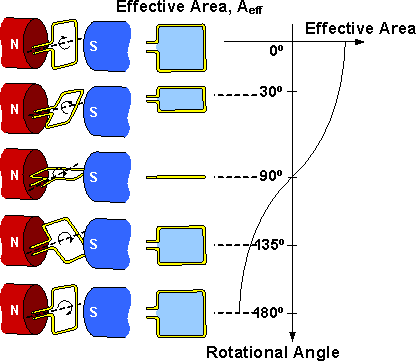Figure 2: Effective Area of the Wire Loop at Different Rotational Angles

The Rate of Change of Φ, dΦ / dt, is the largest at the zero points of the Waveform and is the smallest at the peaks of the Waveform, therefore the Induced Eemf is maximum at the zero points and minimum at the peaks, Figure 3. The Induced Eemf output by the Generator is an AC Voltage and its Waveform is shown in Figure 4.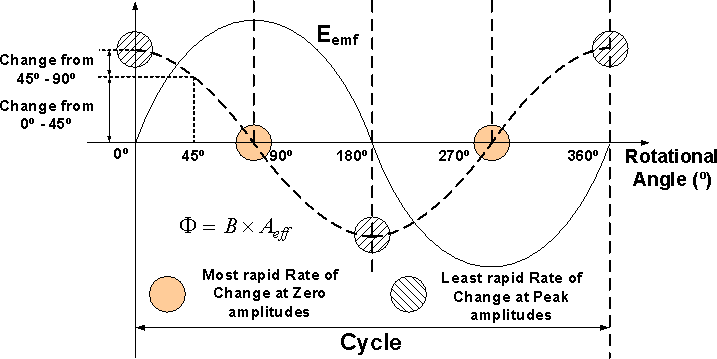Figure 3: Different Rates of Change of the Magnetic Flux at Various Rotational Angles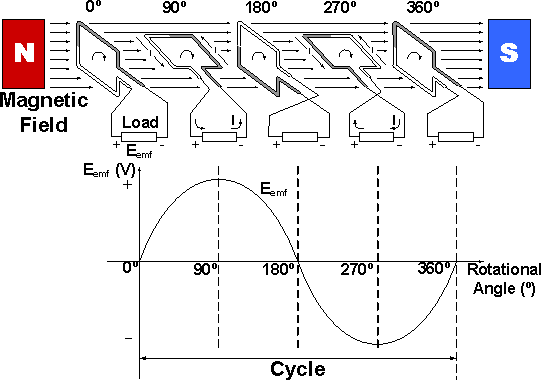Figure 4: Position of the Rotating Wire Coil Plane to the Magnetic Field Direction and the Induced Electromotive Force

AC-Circuits > AC (Alternating Current) Generators